# Differential Calculus NAT Level - 1

## 10 Questions MCQ Test Topic wise Tests for IIT JAM Physics | Differential Calculus NAT Level - 1

Description
Attempt Differential Calculus NAT Level - 1 | 10 questions in 30 minutes | Mock test for IIT JAM preparation | Free important questions MCQ to study Topic wise Tests for IIT JAM Physics for IIT JAM Exam | Download free PDF with solutions
*Answer can only contain numeric values
QUESTION: 1

### If f"(x) > 0 and f'(1) = 0 such that g(x) = f(cot2 x + 2cot x + 2) where 0 < x < π, then g(x) decreasing in (a, b) where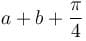is

Solution: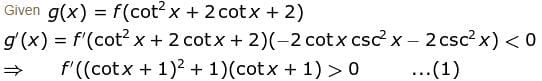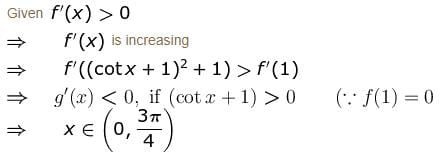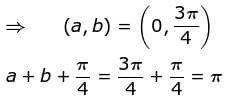*Answer can only contain numeric values
QUESTION: 2

### If f(x) has a maximum or a minimum at a point x0 inside the interval, then f '(x0) equals :

Solution:

It is necessary that at the point of maxima or minima of a function, say ff ' will become zero.

*Answer can only contain numeric values
QUESTION: 3

### If 1" = α radians, then the approximate value of cos 60°1' is given as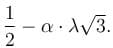Find the value of  λ.

Solution: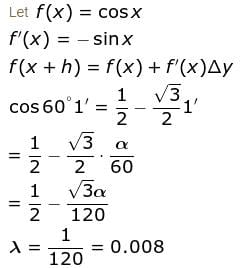*Answer can only contain numeric values
QUESTION: 4

The maximum value of u is, where u = axy2z2 - x2y2z3 - xy3z3 - xy2z4 is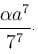Find the value of α.

Solution: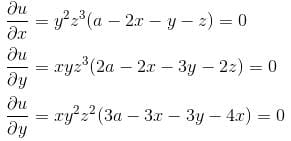Solving, we get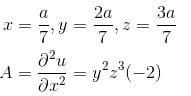Thus, max value is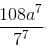*Answer can only contain numeric values
QUESTION: 5

The sum of one number and three times a second number is 60. Find the pair, where product is maximum.

Solution:

Let the two numbers be x & y

⇒ x + 3y = 60 ....(1)

Now, let z = xy = product of two numbers.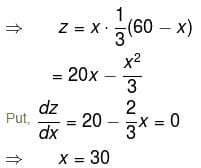Putting x = 30 in (1), we get y = 10 and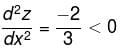Hence, z is maximum when x = 30 and y = 10.

*Answer can only contain numeric values
QUESTION: 6

Let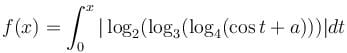be increasing for all real values of x, then range of a is  (α, ∞). Find value of α.

Solution: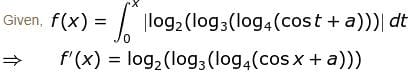Since f(x) is a function so,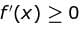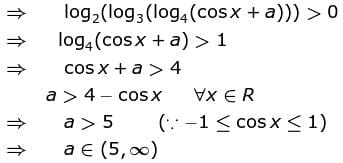Value of α = 5

*Answer can only contain numeric values
QUESTION: 7

The greatest and the least value of the function f(x) = x3 – 18x2 + 96x in the interval [0, 9] are :

Solution:

f(x) = x3 – 18x2 + 96x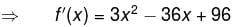= 3(x – 4)(x – 8)
Put f'(x) = 0, we get x = 4, 8
f''(x) = 6x – 36 = 6(x – 6)
which is positive at x = 8 and negative at x = 4

Now, f(0) = 0,  f(8) = 128
f(4) = 160,      f(9) = 135
∴  least value is 0 and greatest value is 160.

*Answer can only contain numeric values
QUESTION: 8

Let f(x, y) = x4 + y4 - 2x2 + 4xy - 2y2 has a minimum at (-√α, √α) and (√α, - √α) Find the value of α.

Solution: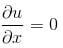⇒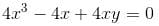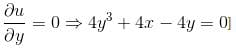Solving, we get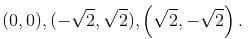r = 12x2 - 4
s = 4
t = 12y2 – 4
at (-√2, √2)
r = 20, s = 4, t = 20
rt - s2 > 0 and r > 0  ∴ minimum

*Answer can only contain numeric values
QUESTION: 9

If the function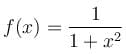is downward concave is (α, β) the [β - α] is

Solution: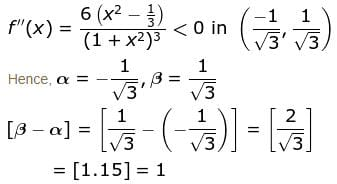∵  [.] greatest integer function

*Answer can only contain numeric values
QUESTION: 10

The minimum value of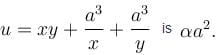Find the value of α.

Solution:

For max. or min value of u,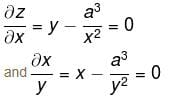Solving these equations, we get
x = y = a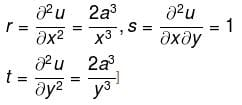at x = ay = a
r = 2, s = 1, t = 2
rt – s2 = 4 – 1 = 3 > 0 and r > 0

Therefore, u is min at x = ay = a and min. value is 3a2.Use Code STAYHOME200 and get INR 200 additional OFF Use Coupon Code